Quick tour of OTclust

1. Introduction

OTclust is an R package for computing a mean partition of an ensemble of clustering results by optimal transport alignment (OTA) and for assessing uncertainty at the levels of both partition and individual clusters. To measure uncertainty, set relationships between clusters in multiple clustering results are revealed. Functions are provided to compute the Covering Point Set (CPS), Cluster Alignment and Points based (CAP) separability, and Wasserstein distance between partitions.

2. Mean partition as an ensemble clustering.

library(OTclust)
data(sim1)

Here, we illustrate the usage of OTclust for an ensemble clustering based on a simulated toy example, sim1, which has 5000 samples, 2 features, and 4 clusters. ensemble( ) generates nbs number of perturbed partitions based on a specified clustering method. For the clustering method, user specified functions or example methods included in package (“kmeans”, “Mclust”, “hclust”, “dbscan”, “PCAreduce”, “HMM-VB”) can be used.

# the number of clusters.
C = 4
# generate an ensemble of perturbed partitions.
# if perturb_method is 1 then perturbed by bootstrap resampling, it it is 0, then perturbed by adding Gaussian noise.
ens.data = ensemble(sim1X, nbs=100, clust_param=C, clustering="kmeans", perturb_method=1) To find a consensus partition, the function otclust( ) searches mean partition by optimal transport alignment (OTA) between the ensemble of partitions. As a return, otclust( ) generates mean partition and its partition-wise and cluster-wise uncertainty statistics. For the detail of return values, refer to help of otclust( ). # find mean partition and uncertainty statistics. ota = otclust(ens.data) # calculate baseline method for comparison. kcl = kmeans(sim1X,C)

# align clustering results for convenience of comparison.
compar = align(cbind(sim1$z,kcl$cluster,ota$meanpart)) lab.match = lapply(compar$weight,function(x) apply(x,2,which.max))
kcl.algnd = match(kcl$cluster,lab.match[]) ota.algnd = match(ota$meanpart,lab.match[])
# plot the result on two dimensional space.
otplot(sim1$X,sim1$z,con=F,title='Truth')   # ground truth
otplot(sim1$X,kcl.algnd,con=F,title='Kmeans') # baseline method otplot(sim1$X,ota.algnd,con=F,title='Mean partition')   # mean partition by OTclust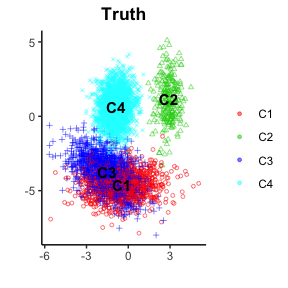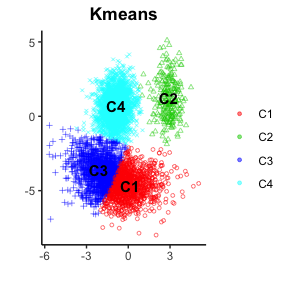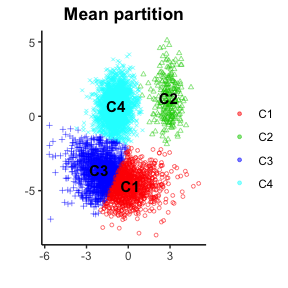3. Uncertainty assessment of clustering results

Here, as cluster-wise uncertainty measures, we briefly introduce the usage of topological relationship statistics of mean partitions, cluster alignment and points based (CAP) separability, and covering point sets (CPS). The detailed definition of the above statistics can be found in . Moveover, if you want to carry out CPS Analysis, please next two sections.

# distance between ground truth and each partition
wassDist(sim1$z,kmeans(sim1$X,C)$cluster) # baseline method #>  0.254152 wassDist(sim1$z,ota$meanpart) # mean partition by OTclust #>  0.2498597 # Topological relationships between mean partition and ensemble clusters t(ota$match)
#>       C1 C2 C3 C4
#> match 82 98 82 82
#> split  0  1  0  0
#> merge  0  0  1  1
#> l.c.  18  1 17 17

# Cluster Alignment and Points based (CAP) separability
ota$cap #> C1 C2 C3 C4 #> C1 0.0000000 0.9121878 0.9993012 1.0000000 #> C2 0.9121878 0.0000000 1.0000000 0.9967047 #> C3 0.9993012 1.0000000 0.0000000 0.9392917 #> C4 1.0000000 0.9967047 0.9392917 0.0000000 # Covering Point Set(CPS) otplot(sim1$X,ota$cps[lab.match[],],legend.labels=c('','CPS'),add.text=F,title='CPS for C1') #> Warning: Removed 2 rows containing missing values (geom_text). otplot(sim1$X,ota$cps[lab.match[],],legend.labels=c('','CPS'),add.text=F,title='CPS for C2') #> Warning: Removed 2 rows containing missing values (geom_text). otplot(sim1$X,ota$cps[lab.match[],],legend.labels=c('','CPS'),add.text=F,title='CPS for C3') #> Warning: Removed 2 rows containing missing values (geom_text). otplot(sim1$X,ota$cps[lab.match[],],legend.labels=c('','CPS'),add.text=F,title='CPS for C4') #> Warning: Removed 2 rows containing missing values (geom_text).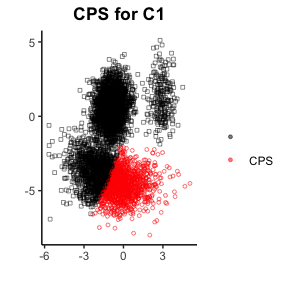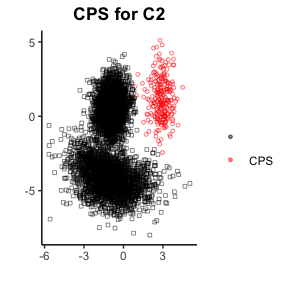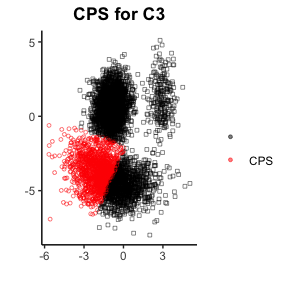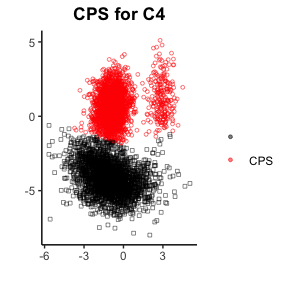The red area of the above plots indicates covering point set (CPS) for each cluster. The detail of the CPS analysis is addressed in the next section. 4. CPS Analysis on selection of visualization methods The functions that are going to be used in this section are visCPS( ), mplot( ) and cplot( ). First, the function visCPS( ) is used for the main computation of the CPS Analysis. The input should include: 1. vlab, which is the visualization coordinates generated by the visualization method that you are going to assess. 2. ref, the true cluster labels of the samples. 3. nEXP, which is optional, the number of perturbed reuslts for CPS Analysis, default 100. Larger the nEXP is, the longer time it will take to compute. # CPS analysis on selection of visualization methods data(vis_pollen) c=visCPS(vis_pollen$vis, vis_pollen$ref) After the computation, we have the return list c, which would be the input of function mplot( ) or cplot( ). The mplot( ) will provide the membership heat map of the required cluster, and the input should be c and the cluster number. The cplot( ) will provide the covering point set plot of the required cluster, and the input should be c and the cluster number. # visualization of the result mplot(c,2) cplot(c,2)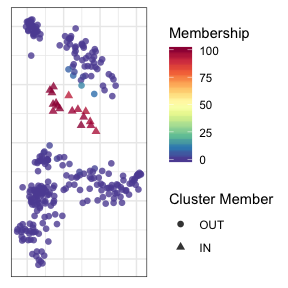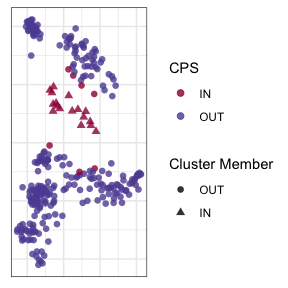Furthermore, if you want to see the statitics, you can simply view the return of visCPS( ): # overall tightness c$tight_all
#>  0.5166624
# cluster-wise tightness
c\$tight
#>                                   1         2 3         4         5
#> Tightness of each cluster 0.2134804 0.7115383 1 0.6092218 0.9272868
#>                                   6        7         8         9        10
#> Tightness of each cluster 0.4363253 0.435473 0.2177813 0.1285714 0.4454768
#>                                  11
#> Tightness of each cluster 0.5581313

5. CPS Analysis on validation of clustering result

In this section, the relevant functions are clustCPS( ), preprocess( ), perturb( ), CPS( ), mplot( ) and cplot( ). For most of the users, you just need to use the clustCPS( ) for the CPS Analysis. It will provide you a lot of choice: For visualization method, you can choose between tsne and umap. You can decide to add the noise before or after the dimension reduction by parameter noi. Also, you can choose to use Kmeans or Mclust as the clustering method. Here is the example of a single cell dataset, choosing to use the log transformation and preprocessing based on the variance, which can reduct the initial dimension of the data set. If you want to use other dimension reduction technique or you need to carry out other preprocession than we proivde, you just need to set l=FALSE, pre=FALSE, dimr=“None”, and then input your processed result as parameter data.

# CPS Analysis on validation of clustering result
data(YAN)
y=clustCPS(YAN, k=7, l=FALSE, pre=FALSE, noi="after", cmethod="kmeans", dimr="PCA", vis="tsne")
#> sigma summary: Min. : 0.323162264525782 |1st Qu. : 0.686532727791371 |Median : 0.840637685950217 |Mean : 0.832540338898672 |3rd Qu. : 0.996223616580691 |Max. : 1.26695806934483 |
#> Epoch: Iteration #100 error is: 14.568977374827
#> Epoch: Iteration #200 error is: 0.485179542650453
#> Epoch: Iteration #300 error is: 0.47141108056016
#> Epoch: Iteration #400 error is: 0.422772036027473
#> Epoch: Iteration #500 error is: 0.422283242087265
#> Epoch: Iteration #600 error is: 0.42178674345771
#> Epoch: Iteration #700 error is: 0.421785891226059
#> Epoch: Iteration #800 error is: 0.421785890574734
#> Epoch: Iteration #900 error is: 0.421785890574302
#> Epoch: Iteration #1000 error is: 0.421785890574302

# visualization of the results
mplot(y,4)
cplot(y,4)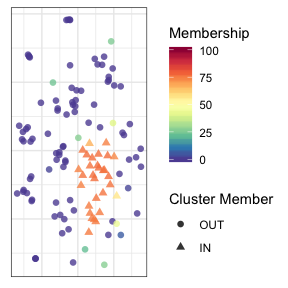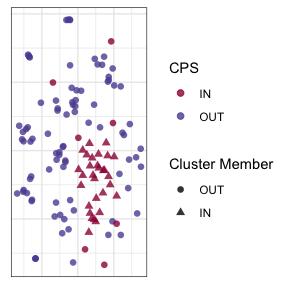If you want to try other clustering method rather than Kmeans or Mclust, you will need to use the function CPS( ). For this function, you need to input several things. First, the reference clustering result, which might be generated by your own clustering method. Second, the 2-dimension visualization coordinates of your samples, which will be further used by mplot( ) or cplot( ). Third, a collection of clustering results in a matrix format, each column represents one clustering result. To get this matrix, you might also want to use the function perturb( ). Suppose the dataset you are going to use for clustering is X, then perturb(X) will give you a perturbed version of it. You can use this perturbed version for clustering to get one clustering result. Repeat this for several times, you will get a collection of clustering results.